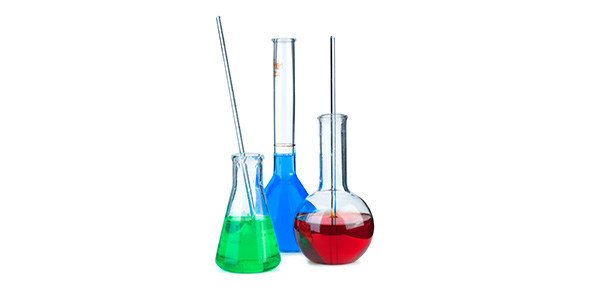# Chemical And Physical Properties

9 Questions | Total Attempts: 1024SettingsAnswer the following questions concerning physical and chemcial properties.

• 1.
What is the universal solvent?
• A.

Alcohol

• B.

Paint thinner

• C.

Water

• D.

Acetone

• 2.
Even though all solid states of other materials are denser than the liquid states of materials, what is a unique property of water?
• A.

It is clear

• B.

Solid water (ice) is less denser than liquid water (ice floats iin water)

• C.

It is tasteless

• D.

It can freeze

• 3.
Which is a list of physical properties of a substance that can be observed or measured without changing the it's identiy?
• A.

Color, shape, texture, odor, melting point, boiling point, density, soluability

• B.

Combusibility, acidity, reactivity

• C.

Acidity, basicity

• D.

Soluablity

• E.

Reactivity, acidity

• 4.
Which chemical property means the ability for a substance to burn?
• A.

Acidity

• B.

Reactivity

• C.

Combustibility

• D.

Basicity

• 5.
What measures the average kinetic energy of the atoms and molecules in matter?
• A.

Liters

• B.

Temperature

• C.

Grams

• D.

Meters

• 6.
What temperature does water turn into ice freezes?
• A.

32 degrees Farenheit

• B.

0 degrees Celsius

• C.

212 degrees Farenhieht

• D.

37 degrees Celcius

• 7.
What is the independent variable of an experiment if you place three of the same types of plants in the same type of soil, then give them the same amounts of water each day, but place them under different colored light?
• A.

The soil

• B.

The plants

• C.

The water

• D.

The lights

• 8.
Referring to question number 7, what is the dependent variable?
• A.

The height the plants grow

• B.

The soil

• C.

The water

• D.

The lights

• 9.
Referring to question number 7, what are the materials in an experiment that are kept the same such as the soil, water, and plants?
• A.

Independent variable

• B.

Constants

• C.

Dependent variable

• D.

Variable

Related TopicsBack to top